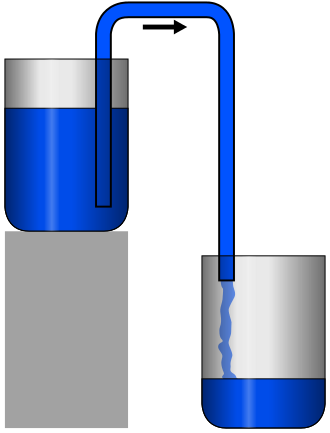top of page
Search
• Shalmali

# Pressure & area: Directly or Inversely ProportionalPressure = Force / Area

An equation that we have been reading, writing using since we were in 8th grade I guess! As shown in the above figure, we apply some force on the apple with the help of a knife. The cutting surface area of the knife is in contact and responsible to cut the apple, which when looked at from the bottom view appears as a line. Force is a vector quantity and we know at what point it is acting. Hence we know, the cutting area. So we can calculate the pressure as pressure = force/area. Hence we can conclude that pressure is inversely proportional to area. It is so simple right?

But wait, you might have observed in fluid dynamics that pressure and area are directly proportional! If you do not know what I am talking about read it here!

What is the exact relationship between pressure and area?

### Pressure a scalar or vector?Pressure does not have any directions hence it is a scalar. And yes, it is a vector in fluid dynamics! It is confusing, right? Let's understand it.

Now consider this. A vehicle is moving. The surrounding air applies pressure, which is called air resistance. Force is a vector. If there is some force would have been acting on the vehicle we could see the point of contact! If we are unable to observe, it should be a scalar quantity. So, air resistance surely is a scalar i.e. Pressure.

How do we calculate the pressure in this case? We need to find a point of contact and the area. So here is what we do. We convert pressure into a vector. How can we do that? We will start using this formula:

Pressure = Force/Area

Force = Pressure * Area

Hence, Pressure Force = Pressure * Area

This is how we convert the scalar pressure to vector pressure and name it pressure force. This pressure force is applied to a point called the Center of Pressure.

That is how by Continuity and Bernoulli's equation, we can say in fluid dynamics that pressure and area are directly proportional.

### Types of Pressure:

Atmospheric Pressure

Atmospheric pressure/barometric pressure is the pressure within the atmosphere of Earth. A standard atmosphere is a unit of pressure defined as 101,325 Pa, which is equivalent to 760mm Hg. The atm unit is roughly equivalent to the mean sea-level atmospheric pressure on Earth; that is, the Earth's atmospheric pressure at sea level is approximately 1 atm.

Guage Pressure/overpressure

Gauge pressure is the pressure relative to atmospheric pressure. Gauge pressure is positive for pressures above atmospheric pressure and negative for pressures below it.

Absolute Pressure

Absolute pressure is measured relative to a full vacuum/absolute zero pressure. Absolute pressure is the sum of gauge pressure and atmospheric pressure.

Vacuum Pressure

Vacuum Pressure is pressure less than atmospheric pressure.

Differential pressure

Differential pressure is essentially the difference in pressure between two given points.

## Siphon Tube

The siphon basically an inverted U-tube or pipe filled with water. The filling of the pipe or tube is simple and many ways it can be done. It is the same as the priming of pumps.### Step 1: Water rising in the input tube.

The inverted U-tube pipe coming out of the higher tank should be shorted in length. The water rising from the tank to the U tube is similar to water rising in the barometer. The liquid in the tube stays there until the tube height is greater than a certain height that depends on the density of the liquid and the pressure of the air outside the tube. Then a near-vacuum forms above the water in the tube, and the water level does not rise further.

if the height of the U-tube of a siphon were too great, the same thing would happen, and a near-vacuum would be created near the top of the U-tube. This would interrupt the continuity of liquid in the tube, and siphon action would stop.

### Step 2: Siphon and Atmospheric pressure.

The atmospheric pressure does not affect the siphoning effect but the cohesive forces between the molecules of the substance do. For example, the siphon phenomenon can not be seen in the sand.

### Step 3: Maintaining the flow

Siphon fluid flow takes place with the help of the Bernoullis Principle and its formula being:

The flow mainly takes place due to gravitational force. For any fluid to flow it is important that there is some pressure difference.The pressure difference between two points in a liquid is equal to ρgH where ρ is the liquid density, g is the acceleration due to gravity and H is the height difference. This is true so long as the liquid has continuity between the two points, that is if a continuous line can be drawn between the points, passing only through the liquid.

## Summary:

1. Pressure is a scalar quantity.

2. Pressure is converted into a vector quantity in fluid dynamics for the purpose of ease.

3. Gauge pressure is positive for pressures above atmospheric pressure and negative for pressures below it. Absolute pressure is measured relative to a full vacuum/absolute zero pressure. Vacuum Pressure is pressure less than atmospheric pressure.

4. The siphon basically an inverted U-tube or pipe filled with water. The filling of the pipe or tube is simple and many ways it can be done.

Want to know more about pressure and fluid dynamics? Subscribe to our blogs!